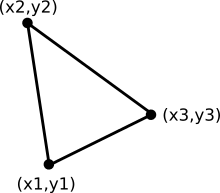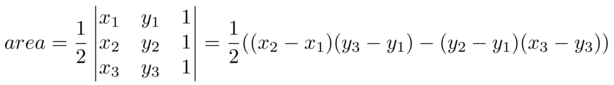Convex Hull

02_clockwise
20161205

# The clockwise function

As you gathered from the outline of the algorithm in the introduction, we need to write a function to decide whether the motion from point 1 to point 2 to a point 3 is clockwise or counterclockwise or collinear. Let's focus on that first.

## Triange areaThe area of a triangle can be calculated from following determinant:It happens to be so that when the area is negative, the motion from point 1 -> 2 -> 3 is a counterclockwise motion. When the calculated area is positive, the motion from point 1 -> 2 -> 3 is a clockwise motion. And when it's zero? Yes, you've guessed it: the points fall on the same line, ie. they are collinear..

## Example Program

The following program is for inspecting whether the clockwise function works as expected: it draws a number of cases, with p1 always at (50,0), p2 at ( 20..80, 50), and p3 random. Depending on clockwise or counterclockwise, the line is drawn in either red or green, to make it possible to visually inspect the correctness.

 ```1 2 3 4 5 6 7 8 9 10 11 12 13 14 15 16 17 18 19 20 21 22 23 24 25 26 27 28 29 ``` ``````#!/usr/bin/python import matplotlib.pyplot as plt import random def x(tp): return tp def y(tp): return tp # return 'g', 'b','r' respectively if counterclockwise, collinear, or clockwise def clockwise(pt1, pt2, pt3): half_area = (x(pt2)-x(pt1))*(y(pt3)-y(pt1))-(y(pt2)-y(pt1))*(x(pt3)-x(pt1)) rv='b' # collinear if half_area<0: rv='r' # clockwise elif half_area>0: rv='g' # counter clockwise return rv p1=(50.0,0.0) for i in range(7): p2= ( 50+(i-3)*10, 50) sgn=random.sample([-1,1],1) p3= ( 20+random.randint(1,60), 50+ sgn*(10 + random.randint(1,30)) ) col=clockwise(p1,p2,p3) plt.plot(*zip(*[p1,p2,p3]), color=col, linewidth=2.0, marker='o') plt.show() ``````

Note: the x() and y() functions are convenience functions to get the x and y element from a point (a tuple with 2 elements), because this formula is easier to read ..

``half_area = (x(pt2)-x(pt1))*(y(pt3)-y(pt1))-(y(pt2)-y(pt1))*(x(pt3)-x(pt1))``

.. than this one ..

``half_area = (pt2-pt1)*(pt3-pt1)-(pt2-pt1)*(pt3)-pt1)``

### Example Plot

The following is a plot generated by the above program. When inspecting clockwise or not, start with P1 at the bottom, and choose a line going up to P2, and continue to P3. Is it a clockwise motion? The line should be in red then.Notes by Data Munging Ninja. Generated on nini:sync/20151223_datamungingninja/convexhull at 2016-12-26 08:22